# graph

(redirected from graphed)
Also found in: Dictionary, Thesaurus, Medical, Legal, Financial.

## graph,

figure that shows relationships between quantities. The graph of a function y=f (x) is the set of points with coordinates [x, f (x)] in the xy-plane, when x and y are numbers. A similar definition can be given for functions involving more general kinds of variables. In mathematics interest is almost exclusively in line graphs and what these reveal about the functions they represent. Statistics makes extensive use of both line graphs and bar graphs, in which the lengths of the various bars show the quantities to be compared. Graph is also a mathematical term used in combinatorics to designate a geometric object consisting of vertices and edges (joining pairs of vertices). Such objects have been studied considerably in recent years because of the applicability to such diverse fields as computer networks, game theory, and social psychology.

## Graph

a geometric representation of functional relationships with the aid of a line in a plane. For example, Figure 1 shows the change in atmospheric pressure with time. Graphs are used for the visual representation of functional relation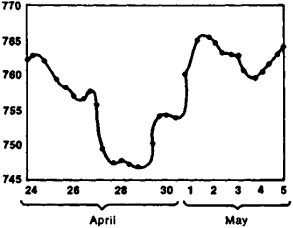Figure 1. Graph showing change in atmospheric pressure (in mm Hg) for the period April 24 to May 5

ships and as a visual aid for the study of such relationships; they are also used for a rapid determination of the value of functions from the values of the independent variables. There are many types of graphs. The type of a graph depends on which coordinate system (in a plane) is used as the basis for it. Once the coordinate system is chosen, the graph of the function βξ) is simply a set of points (or the so-called locus) the coordinates of which satisfy the equation y = βξ). In most cases graphs are plotted using the rectangular Cartesian coordinates. Figure 2 shows the graph of the function y = x2 (a parabola). Figure 3 shows the graph of the function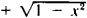which is a semicircle originating at the point (-1, 0) and terminating at the point (+ 1, 0).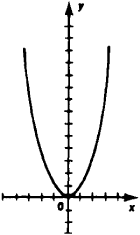Figure 2. Graph of the function Γ = x2 (Parabola)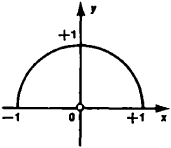Figure 3.

Note to Figure 3. Graph of the function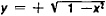(semicircle)

In a rectangular coordinate system the scale is the same for both axes. In practice, however, this inconvenient restriction is disregarded, and different scales for each axis are selected such that the best possible utilization of the graph paper can be achieved. Graphs based on other coordinate systems are also used, for instance, the polar coordinate system. This system is particularly convenient when representing the functions of an angular argument. (Figure 4 shows graphs, in a polar coordinate system, of the distribution of luminous intensity emitted in varying directions by three different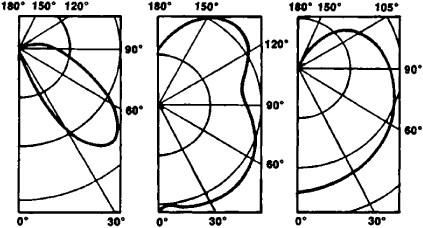Figure 4. Polar diagram of luminous intensity of three types of arc lamps

types of arc lamps.) Sometimes it is expedient to simplify a graph by using some appropriate function of the variables x and y for the coordinates of a point. For instance, if a point with Cartesian coordinates (log x, log y) corresponds to the values of the independent variable and the function—values (x, y)—the graphs of the function y = xn turn out to be straight lines for any value of the exponent n (see Figure 5). Semilogarithmic graph paper and logarithmic graph paper are used to quickly plot such a graph.

If the graph is a straight line, it can be constructed from two given points using a ruler; if it is an arc of a circle, it can be drawn from three given points using a compass. In all other cases it becomes necessary to plot a sufficiently large number of given points on paper and then to draw freehand the line connecting these points. This procedure is always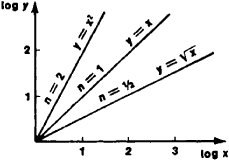Figure 5. Graph of the function y = xn for n = ½, 1, 2. Log x and Log y are shown on coordinate axes.

somewhat arbitrary and is justified only if a continuous function is assumed. Functions that are not only continuous but also sufficiently “smooth” can be plotted quite accurately with some experience. (A smooth function is one whose second and third derivatives do not change too drastically with a change in the independent variable.) If the graphs of the functions y = Φ1 (x) and y = Φ2(x) are all plotted on the same diagram, their points of intersection will determine the roots of the equation Φ1 (x) = Φ2(x)

There are numerous recording devices that automatically plot graphs of an observed functional relationship on paper, bypassing its analytic expression (for instance, a barograph plots a graph of atmospheric pressure as a function of time). Diagrams are frequently used for the graphic representation of a relationship between quantities. In economics and in production management, standard graphs (charts) and planning charts are widely used, as are organizational charts showing the organizational relationships (for instance, the administrative scheme of an industrial plant). In many types of problems it is convenient to simultaneously view several different functions on the same diagram. A typical example of this kind of graph is the traffic chart used in transportation.

## graph

[graf]
(mathematics)
The planar object, formed from points and line segments between them, used in the study of circuits and networks.
The graph of a function ƒ is the set of all ordered pairs [x,ƒ(x)], where x is in the domain of ƒ.
The set of all points that satisfy a particular equation, inequality, or system of equations or inequalities.

## graph

Maths
1. a drawing depicting the relation between certain sets of numbers or quantities by means of a series of dots, lines, etc., plotted with reference to a set of axes
2. a drawing depicting a functional relation between two or three variables by means of a curve or surface containing only those points whose coordinates satisfy the relation
3. a structure represented by a diagram consisting of points (vertices) joined by lines (edges)

## graph

(mathematics)
A collection of nodes and edges.

See also connected graph, degree, directed graph, Moore bound, regular graph, tree.

## graph

(graphics)
A visual representation of algebraic equations or data.

## graph

A visual representation of information. See business graphics.
References in periodicals archive ?
In the other eight cases the financial performance of the company with the lowest growth rate was inaccurately graphed, but the graphs of the financial performance of the medium growth rate company were accurately drawn.
When the lowest growth rate company's financial data was graphed accurately, participants correctly chose not to invest in that company 96.
36 percent of the time when graphs of its financial performance were graphed correctly.
14 percent of the time when its financial performance was accurately graphed, and 86.

Site: Follow: Share:
Open / Close